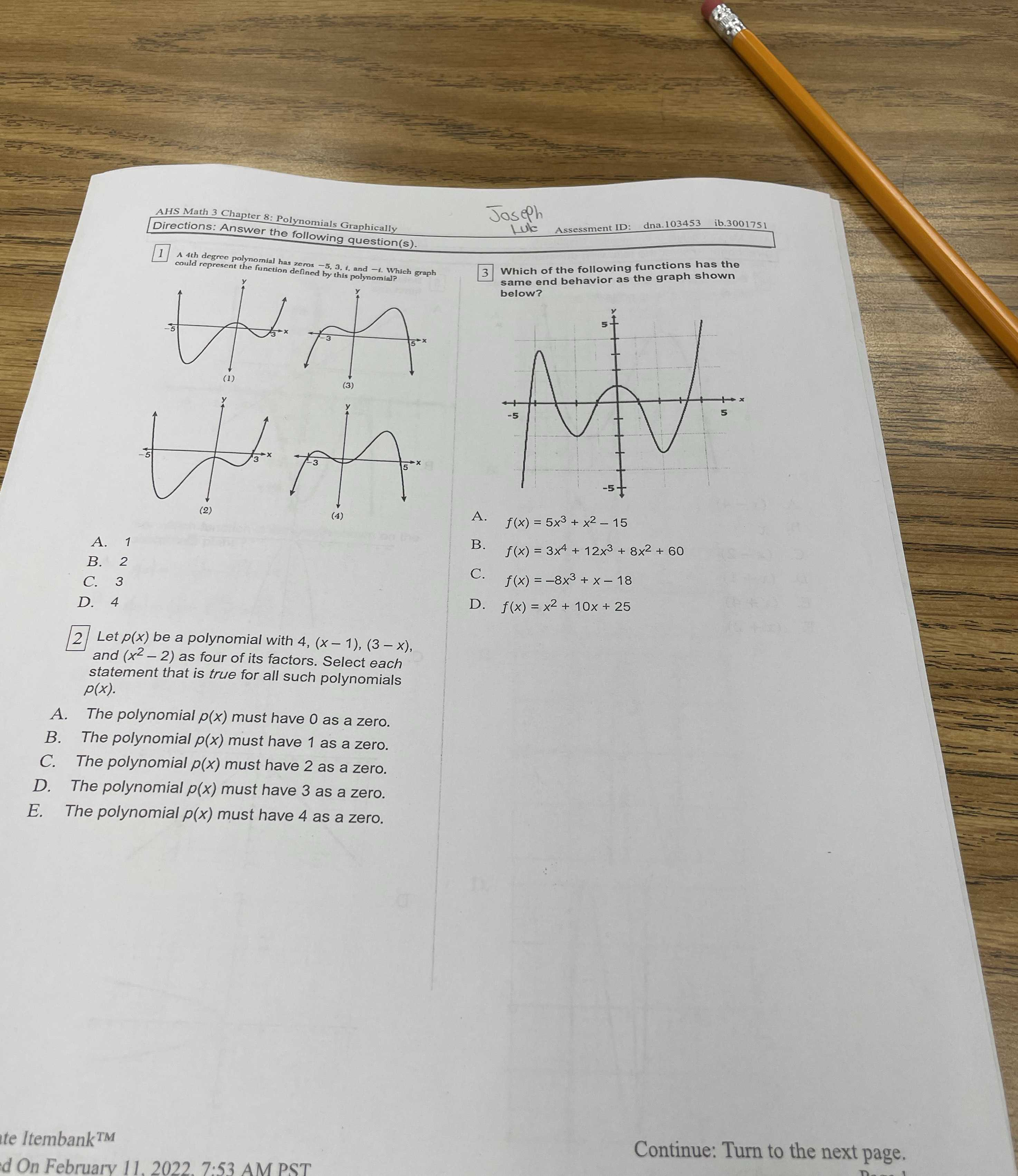### Still have math questions?

Algebra
Question1. A 4th degree polynomial has zeros $$- 5,3 ,$$ , and $$- 1$$ . Which graph could represent the function defincd by this polynomial?

A. $$1$$ B. $$2$$ C. $$3$$ D. $$4$$

2. Let $$p ( x )$$ be a polynomial with $$4 , ( x - 1 ) , ( 3 - x )$$ , and $$( x ^ { 2 } - 2 )$$ as four of its factors. Select each statement that is true for all such polynomials $$p ( x )$$ .

A. The polynomial $$p ( x )$$ must have $$0$$ as a zero.

$$B$$ . The polynomial $$p ( x )$$ must have $$1$$ as a zero.

$$C$$ . The polynomial $$p ( x )$$ must have $$2$$ as a zero.

$$D$$ . The polynomial $$p ( x )$$ must have $$3$$ as a zero.

$$E$$ . The polynomial $$p ( x )$$ must have $$4$$ as a zero.

$$3$$ Which of the following functions has the same end behavior as the graph shown below?

A. $$f ( x ) = 5 x ^ { 3 } + x ^ { 2 } - 15$$

B. $$f ( x ) = 3 x ^ { 4 } + 12 x ^ { 3 } + 8 x ^ { 2 } + 60$$

C. $$f ( x ) = - 8 x ^ { 3 } + x - 18$$

D. $$f ( x ) = x ^ { 2 } + 10 x + 25$$